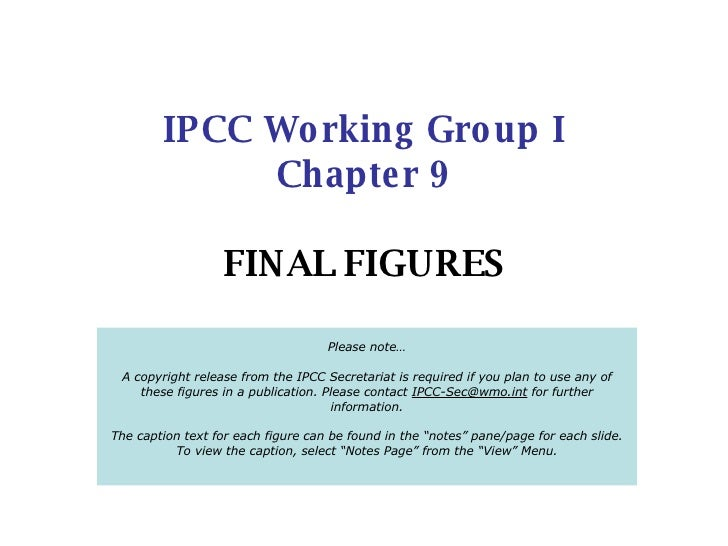Successfully reported this slideshow.
We use your LinkedIn profile and activity data to personalize ads and to show you more relevant ads. You can change your ad preferences anytime.Upcoming SlideShare
×

香港六合彩 &raquo; SlideShare

803 views

Published on

• Full Name
Comment goes here.

Are you sure you want to Yes No
Your message goes here• Be the first to comment

• Be the first to like this

香港六合彩 &raquo; SlideShare

1. 1. IPCC Working Group I Chapter 9 FINAL FIGURES Please note… A copyright release from the IPCC Secretariat is required if you plan to use any of these figures in a publication. Please contact [email_address] for further information. The caption text for each figure can be found in the “notes” pane/page for each slide. To view the caption, select “Notes Page” from the “View” Menu.
2. 2. Figure 9.1
3. 3. Figure 9.2
4. 4. Figure 9.3
5. 5. Figure 9.4
6. 6. Figure 9.5
7. 7. Figure 9.6
8. 8. Figure 9.7
9. 9. Figure 9.8
10. 10. Figure 9.9
11. 11. Figure 9.10
12. 12. Figure 9.11
13. 13. Figure 9.12
14. 14. Figure 9.13
15. 15. Figure 9.14
16. 16. Figure 9.15
17. 17. Figure 9.16
18. 18. Figure 9.17
19. 19. Figure 9.18
20. 20. Figure 9.19
21. 21. Figure 9.20
22. 22. Figure 9.21
23. 23. FAQ 9.1, Figure 1
24. 24. FAQ 9.2, Figure 1